• tensorflow均方误差损失函数 引用API：tensorflow.kreas.losses.MSE 均方误差（Mean Square Erroe）是回归问题最常用的损失函数，回归问题解决的是对具体数值的预测，而不是预测某个事先定义好的分类 则，...
tensorflow均方误差损失函数

引用API：tensorflow.kreas.losses.MSE

均方误差（Mean Square Erroe）是回归问题最常用的损失函数，回归问题解决的是对具体数值的预测，而不是预测某个事先定义好的分类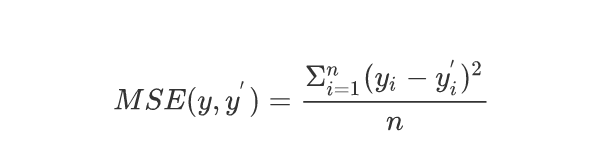则，lose_mean = tf.reduce_mean(tf.square(y_, y)) #y_为真实值，y_为计算出的预测值

tensorflow均方误差损失函数预测酸奶价格
import tensorflow as tf
import numpy as np

seed = 23456 #定义种子数值

rdm = np.random.RandomState(seed) #生成[0, 1)之间的小数,由于固定seed数值，每次生成的数值都一样

x = rdm.rand(32, 2) #生成32行2列的输入特征x

np.set_printoptions(threshold=6) #概略显示

print("32行2列的特征xs为：", x)  # 打印输入特征，概略显示5行

y_ = [[x1 + x2 + (rdm.rand()  / 10.0 - 0.05)] for (x1, x2) in x] #从x中取出x1,x2相加，并且加上-0.05到+0.05随机噪声，得到标准答案y_

x = tf.cast(x, dtype = tf.float32) #转换x数据类型

print("转换数据类型后x:", x) #打印转换书籍类型后的x

w1 = tf.Variable(tf.random.normal([2, 1], stddev = 1, seed = 1)) #初始化参数w1，生成两行一列的可训练参数

print("w1为：", w1)

epoch = 15000 #迭代次数为15000次

lr = 0.002 #学习率为0.002

for epoch in range(epoch):
y = tf.matmul(x, w1) #求前向传播计算结果y
loss_mse = tf.reduce_mean(tf.square(y_ - y)) #求均方误差loss_mean
# print("y为：", y)
# print("loss_mean为：", loss_mse)

if epoch % 500 == 0: #每迭代500轮
print("After %d training steps, w1 is " % epoch) #每迭代500轮打印当前w1参数
print(w1.numpy(), "\n") #打印当前w1参数

print("Final w1 is :", w1.numpy()) #打印最终w1


结果为：

E:\Anaconda3\envs\TF2\python.exe C:/Users/Administrator/PycharmProjects/untitled8/酸奶价格预测.py 32行2列的特征xs为： [[0.32180029 0.32730047]  [0.92742231 0.31169778]  [0.16195411 0.36407808]  ...  [0.83554249 0.59131388]  [0.2829476  0.05663651]  [0.2916721  0.33175172]] 转换数据类型后x: tf.Tensor( [[0.3218003  0.32730046]  [0.9274223  0.31169778]  [0.1619541  0.36407807]  ...  [0.8355425  0.5913139 ]  [0.2829476  0.05663651]  [0.29167208 0.33175173]], shape=(32, 2), dtype=float32) w1为： <tf.Variable 'Variable:0' shape=(2, 1) dtype=float32, numpy= array([[-0.8113182],        [ 1.4845988]], dtype=float32)> After 0 training steps, w1 is  [[-0.8093924]  [ 1.4857364]]
After 500 training steps, w1 is  [[-0.17545831]  [ 1.7493846 ]]
After 1000 training steps, w1 is  [[0.12224181]  [1.7290779 ]]
After 1500 training steps, w1 is  [[0.30012047]  [1.639089  ]]
After 2000 training steps, w1 is  [[0.42697424]  [1.5418574 ]]
After 2500 training steps, w1 is  [[0.5261725]  [1.4540646]]
After 3000 training steps, w1 is  [[0.6068525]  [1.378847 ]]
After 3500 training steps, w1 is  [[0.6734775]  [1.3155416]]
After 4000 training steps, w1 is  [[0.72881055]  [1.2626004 ]]
After 4500 training steps, w1 is  [[0.77486306]  [1.2184267 ]]
After 5000 training steps, w1 is  [[0.81322  ]  [1.1816002]]
After 5500 training steps, w1 is  [[0.8451773]  [1.1509084]]
After 6000 training steps, w1 is  [[0.87180513]  [1.1253314 ]]
After 6500 training steps, w1 is  [[0.8939932]  [1.1040187]]
After 7000 training steps, w1 is  [[0.9124815]  [1.0862588]]
After 7500 training steps, w1 is  [[0.9278873]  [1.07146  ]]
After 8000 training steps, w1 is  [[0.94072455]  [1.0591285 ]]
After 8500 training steps, w1 is  [[0.9514216]  [1.0488532]]
After 9000 training steps, w1 is  [[0.96033514]  [1.040291  ]]
After 9500 training steps, w1 is  [[0.9677624]  [1.0331562]]
After 10000 training steps, w1 is  [[0.9739516]  [1.0272108]]
After 10500 training steps, w1 is  [[0.97910905]  [1.0222566 ]]
After 11000 training steps, w1 is  [[0.98340666]  [1.0181286 ]]
After 11500 training steps, w1 is  [[0.98698765]  [1.0146885 ]]
After 12000 training steps, w1 is  [[0.98997146]  [1.0118226 ]]
After 12500 training steps, w1 is  [[0.9924576]  [1.0094334]]
After 13000 training steps, w1 is  [[0.99452955]  [1.0074434 ]]
After 13500 training steps, w1 is  [[0.9962558]  [1.0057855]]
After 14000 training steps, w1 is  [[0.997694 ]  [1.0044047]]
After 14500 training steps, w1 is  [[0.9988928]  [1.0032523]]
Final w1 is : [[0.99988985]  [1.0022951 ]]
Process finished with exit code 0


展开全文机器学习 tensorflow 深度学习 python 神经网络
• 比如对于一个4分类问题，期望输出应该为g0=[0,1,0,0]，实际输出为g1=[0.2,0.4,0.4,0]，计算g1与g0之间的差异所使用的方法，就是损失函数，分类问题中常用损失函数是交叉熵。 交叉熵（cross entropy）描述的是两个...
交叉熵的对比二次方损失
https://blog.csdn.net/u012162613/article/details/44239919
这篇没看懂
https://blog.csdn.net/u014313009/article/details/51043064

分类问题中，预测结果是（或可以转化成）输入样本属于n个不同分类的对应概率。比如对于一个4分类问题，期望输出应该为 g0=[0,1,0,0] ，实际输出为 g1=[0.2,0.4,0.4,0] ，计算g1与g0之间的差异所使用的方法，就是损失函数，分类问题中常用损失函数是交叉熵。
交叉熵（cross entropy）描述的是两个概率分布之间的距离，距离越小表示这两个概率越相近，越大表示两个概率差异越大。对于两个概率分布 p 和 q ，使用 q 来表示 p 的交叉熵为：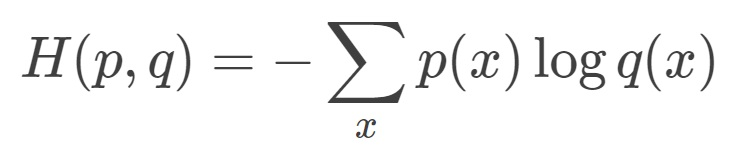由公式可以看出来，p 与 q 之间的交叉熵 和 q 与 p 之间的交叉熵不是等价的。上式表示的物理意义是使用概率分布 q 来表示概率分布 p 的困难程序，q 是预测值，p 是期望值。

神经网络的输出，也就是前向传播的输出可以通过Softmax回归变成概率分布，之后就可以使用交叉熵函数计算损失了。
交叉熵一般会跟Softmax一起使用，在tf中对这两个函数做了封装，就是 tf.nn.softmax_cross_entropy_with_logits 函数，可以直接计算神经网络的交叉熵损失。
cross_entropy = tf.nn.softmax_cross_entropy_with_logits(y, y_)

其中 y 是网络的输出，y_ 是期望输出。

针对分类任务中，正确答案往往只有一个的情况，tf提供了更加高效的 tf.nn.sparse_softmax_cross_entropy_with_logits 函数来求交叉熵损失。

均方误差

与分类任务对应的是回归问题，回归问题的任务是预测一个具体的数值，例如雨量预测、股价预测等。回归问题的网络输出一般只有一个节点，这个节点就是预测值。这种情况下就不方便使用交叉熵函数求损失函数了。
回归问题中常用的损失函数式均方误差(MSE,mean squared error)，定义如下：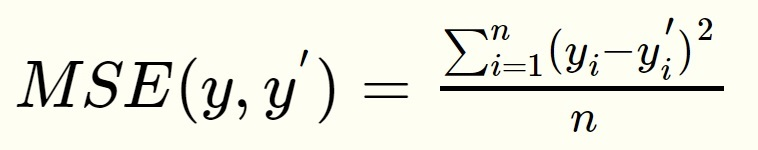均方误差的含义是求一个batch中n个样本的n个输出与期望输出的差的平方的平均值。
tf中实现均方误差的函数为：
mse = tf.reduce_mean(tf.square(y_ - y))

在有些特定场合，需要根据情况自定义损失函数，例如对于非常重要场所的安检工作，把一个正常物品错识别为危险品和把一个危险品错识别为正常品的损失显然是不一样的，宁可错判成危险品，不能漏判一个危险品，所以就要在定义损失函数的时候就要区别对待，对漏判加一个较大的比例系数。在tf中可以通过以下函数自定义：

loss = tf.reduce_sum(tf.select(tf.greater(v1,v2),loss1,loss2))

https://blog.csdn.net/dcrmg/article/details/80010342
展开全文• 一、均方误差损失函数 对于回归问题，最常用的损失函数就是均方误差损失函数（MSE） 定义如下： 其中yi为输出中第i个数据的答案值，yi‘ 就是神经网络给出的预测值。因此最小化该函数就是优化的目标。 通过最小...
一、均方误差损失函数
对于回归问题，最常用的损失函数就是均方误差损失函数（MSE） 定义如下：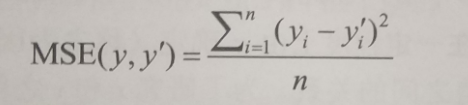其中yi为输出中第i个数据的答案值，yi‘ 就是神经网络给出的预测值。因此最小化该函数就是优化的目标。 通过最小二乘法，可以获得让MSE最小的数值。令偏导分别为0，则会有一个方程组，方程组化简后，会得到一个由n，yi，xi表达的式子，这样就可以获得相应的方程组，方便计算机求解。 最终会转化成下图的形式：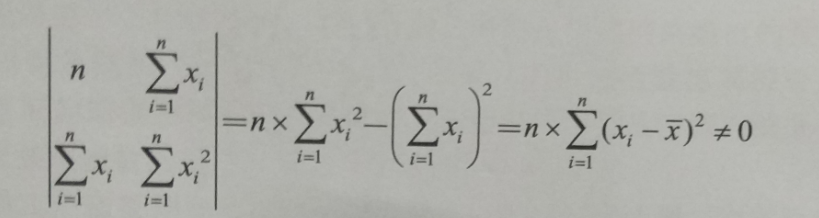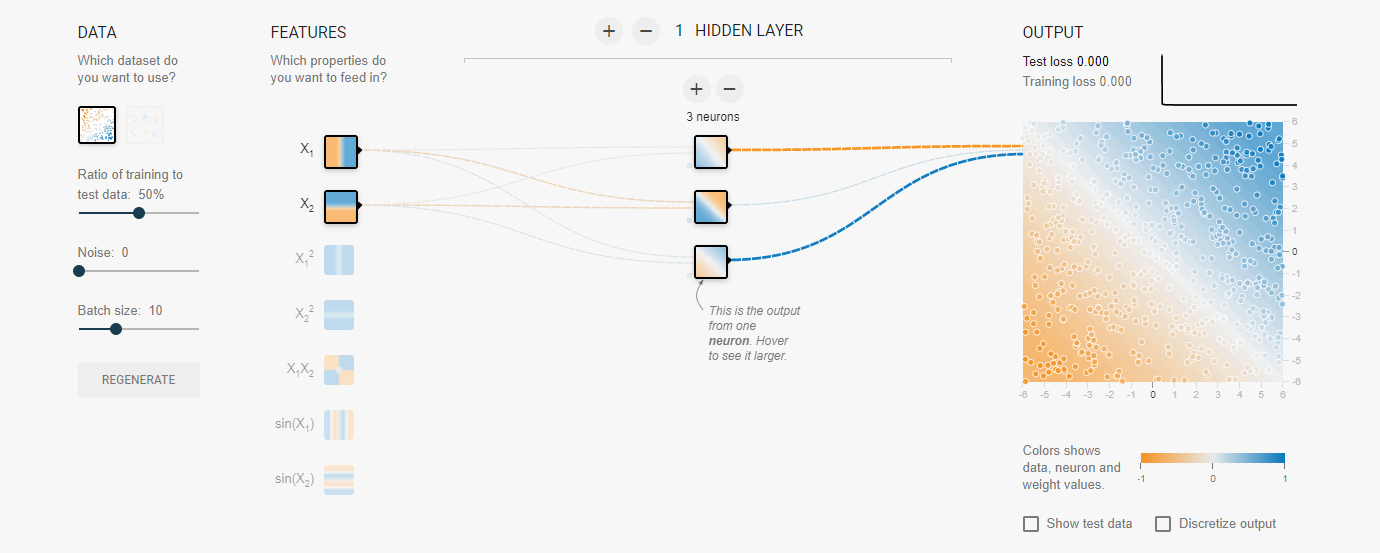二、自定义损失函数
举例说明自定义损失函数： 若预测某商品的出货量，每比实际的多出1个则损失2元，每比实际的少1个则损失5元。 因此它的损失函数可以如下表达：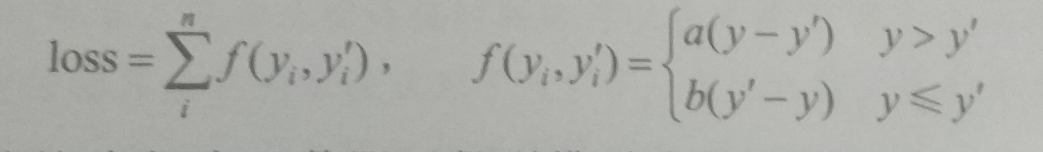最小化上述的损失函数，则可以给出最佳的出货量。 在tensorflow中可以通过 where（）和greater（）函数实现上述的损失函数。
loss  =tf.reduce_sun(tf.where(tf.greater(y,y_),(y-y_)*a,(y_-y)*b))
#greater()输入两个张量（同维度），此函数会比较两个张量对应位置的大小，第一个大于第二个则返回True
#where（）为选择功能，第一个为选择条件。是一个bool张量，当为True时会选择第二个进行执行，否则执行第三个

例程如下：
import tensorflow as tf
tf.compat.v1.disable_eager_execution()
a = tf.constant([1.0,2.0,3.0,4.0,])
b = tf.constant([6.0,5.0,4.0,3.0,])
with tf.compat.v1.Session() as sess:
print(tf.greater(a,b))
print(tf.where(tf.greater(a,b),a,b))
print(sess.run(tf.greater(a,b)))
print(sess.run(tf.where(tf.greater(a,b),a,b)))

输出结果：
......
......
......
Skipping registering GPU devices...
2020-06-30 15:56:49.766413: I tensorflow/core/platform/cpu_feature_guard.cc:143] Your CPU supports instructions that this TensorFlow binary was not compiled to use: AVX2
2020-06-30 15:56:49.778833: I tensorflow/compiler/xla/service/service.cc:168] XLA service 0x22aeb00e460 initialized for platform Host (this does not guarantee that XLA will be used). Devices:
2020-06-30 15:56:49.779383: I tensorflow/compiler/xla/service/service.cc:176]   StreamExecutor device (0): Host, Default Version
2020-06-30 15:56:49.779798: I tensorflow/core/common_runtime/gpu/gpu_device.cc:1102] Device interconnect StreamExecutor with strength 1 edge matrix:
2020-06-30 15:56:49.780145: I tensorflow/core/common_runtime/gpu/gpu_device.cc:1108]
Tensor("Greater:0", shape=(4,), dtype=bool)
Tensor("SelectV2:0", shape=(4,), dtype=float32)
[False False False  True]
[6. 5. 4. 4.]

展开全文深度学习 tensorflow 机器学习 python
• 文章目录交叉熵损失与均方误差损失损失函数角度softmax反向传播角度参考 交叉熵损失与均方误差损失 常规分类网络最后的softmax层如下图所示，传统机器学习方法以此类比， 一共有KKK类，令网络的输出为[y^1,…,y^K]...


文章目录
交叉熵损失与均方误差损失损失函数角度softmax反向传播角度参考

博客：
blog.shinelee.me |
博客园 |
CSDN

交叉熵损失与均方误差损失
常规分类网络最后的softmax层如下图所示，传统机器学习方法以此类比，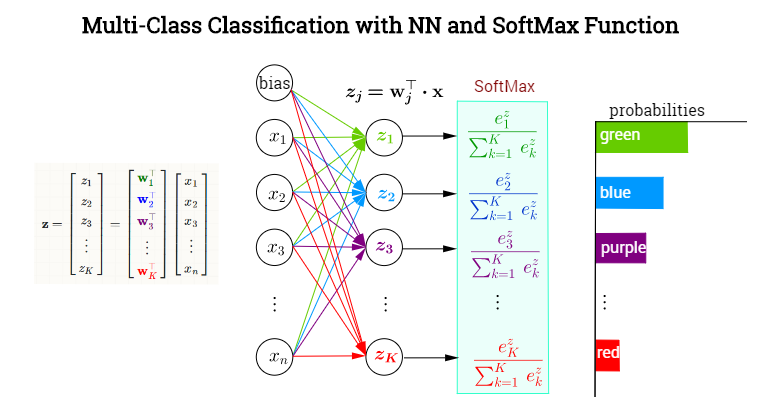一共有

K

K

类，令网络的输出为

[

y

^

1

,

…

,

y

^

K

]

[\hat{y}_1,\dots, \hat{y}_K]

，对应每个类别的概率，令label为

[

y

1

,

…

,

y

K

]

[y_1, \dots, y_K]

。对某个属于

p

p

类的样本，其label中

y

p

=

1

y_p=1

，

y

1

,

…

,

y

p

−

1

,

y

p

+

1

,

…

,

y

K

y_1, \dots, y_{p-1}, y_{p+1}, \dots, y_K

均为0。
对这个样本，交叉熵（cross entropy）损失为

L

=

−

(

y

1

log

⁡

y

^

1

+

⋯

+

y

K

log

⁡

y

^

K

)

=

−

y

p

log

⁡

y

^

p

=

−

log

⁡

y

^

p

\begin{aligned}L &= - (y_1 \log \hat{y}_1 + \dots + y_K \log \hat{y}_K) \\&= -y_p \log \hat{y}_p \\ &= - \log \hat{y}_p\end{aligned}

**均方误差损失（mean squared error，MSE）**为

L

=

(

y

1

−

y

^

1

)

2

+

⋯

+

(

y

K

−

y

^

K

)

2

=

(

1

−

y

^

p

)

2

+

(

y

^

1

2

+

⋯

+

y

^

p

−

1

2

+

y

^

p

+

1

2

+

⋯

+

y

^

K

2

)

\begin{aligned}L &= (y_1 - \hat{y}_1)^2 + \dots + (y_K - \hat{y}_K)^2 \\&= (1 - \hat{y}_p)^2 + (\hat{y}_1^2 + \dots + \hat{y}_{p-1}^2 + \hat{y}_{p+1}^2 + \dots + \hat{y}_K^2)\end{aligned}

则

m

m

个样本的损失为

ℓ

=

1

m

∑

i

=

1

m

L

i

\ell = \frac{1}{m} \sum_{i=1}^m L_i

对比交叉熵损失与均方误差损失，只看单个样本的损失即可，下面从两个角度进行分析。
损失函数角度
损失函数是网络学习的指挥棒，它引导着网络学习的方向——能让损失函数变小的参数就是好参数。
所以，损失函数的选择和设计要能表达你希望模型具有的性质与倾向。
对比交叉熵和均方误差损失，可以发现，两者均在

y

^

=

y

=

1

\hat{y} = y = 1

时取得最小值0，但在实践中

y

^

p

\hat{y}_p

只会趋近于1而不是恰好等于1，在

y

^

p

<

1

\hat{y}_p < 1

的情况下，
交叉熵只与label类别有关，

y

^

p

\hat{y}_p

越趋近于1越好均方误差不仅与

y

^

p

\hat{y}_p

有关，还与其他项有关，它希望

y

^

1

,

…

,

y

^

p

−

1

,

y

^

p

+

1

,

…

,

y

^

K

\hat{y}_1, \dots, \hat{y}_{p-1}, \hat{y}_{p+1}, \dots, \hat{y}_K

越平均越好，即在

1

−

y

^

p

K

−

1

\frac{1-\hat{y}_p}{K-1}

时取得最小值
分类问题中，对于类别之间的相关性，我们缺乏先验。
虽然我们知道，与“狗”相比，“猫”和“老虎”之间的相似度更高，但是这种关系在样本标记之初是难以量化的，所以label都是one hot。
在这个前提下，均方误差损失可能会给出错误的指示，比如猫、老虎、狗的3分类问题，label为

[

1

,

0

,

0

]

[1, 0, 0]

，在均方误差看来，预测为

[

0.8

,

0.1

,

0.1

]

[0.8, 0.1, 0.1]

要比

[

0.8

,

0.15

,

0.05

]

[0.8, 0.15, 0.05]

要好，即认为平均总比有倾向性要好，但这有悖我们的常识。
而对交叉熵损失，既然类别间复杂的相似度矩阵是难以量化的，索性只能关注样本所属的类别，只要

y

^

p

\hat{y}_p

越接近于1就好，这显示是更合理的。
softmax反向传播角度
softmax的作用是将

(

−

∞

,

+

∞

)

(-\infty, +\infty)

的几个实数映射到

(

0

,

1

)

(0,1)

之间且之和为1，以获得某种概率解释。
令softmax函数的输入为

z

z

，输出为

y

^

\hat{y}

，对结点

p

p

有，

y

^

p

=

e

z

p

∑

k

=

1

K

e

z

k

\hat{y}_p = \frac{e^{z_p}}{\sum_{k=1}^K e^{z_k}}

y

^

p

\hat{y}_p

不仅与

z

p

z_p

有关，还与

{

z

k

∣

k

≠

p

}

\{z_k | k\neq p\}

有关，这里仅看$z_p$，则有

∂

y

^

p

∂

z

p

=

y

^

p

(

1

−

y

^

p

)

\frac{\partial \hat{y}_p}{\partial z_p} = \hat{y}_p(1-\hat{y}_p)

y

^

p

\hat{y}_p

为正确分类的概率，为0时表示分类完全错误，越接近于1表示越正确。根据链式法则，按理来讲，对与

z

p

z_p

相连的权重，损失函数的偏导会含有

y

^

p

(

1

−

y

^

p

)

\hat{y}_p(1-\hat{y}_p)

这一因子项，

y

^

p

=

0

\hat{y}_p = 0

时分类错误，但偏导为0，权重不会更新，这显然不对——分类越错误越需要对权重进行更新。
对交叉熵损失，

∂

L

∂

y

^

p

=

−

1

y

^

p

\frac{\partial L}{\partial \hat{y}_p} = -\frac{1}{\hat{y}_p}

则有

∂

L

∂

z

^

p

=

∂

L

∂

y

^

p

⋅

∂

y

^

p

∂

z

p

=

y

^

p

−

1

\frac{\partial L}{\partial \hat{z}_p} = \frac{\partial L}{\partial \hat{y}_p} \cdot \frac{\partial \hat{y}_p}{\partial z_p} = \hat{y}_p - 1

恰好将

y

^

p

(

1

−

y

^

p

)

\hat{y}_p(1-\hat{y}_p)

中的

y

^

p

\hat{y}_p

消掉，避免了上述情形的发生，且

y

^

p

\hat{y}_p

越接近于1，偏导越接近于0，即分类越正确越不需要更新权重，这与我们的期望相符。
而对均方误差损失，

∂

L

∂

y

^

p

=

−

2

(

1

−

y

^

p

)

=

2

(

y

^

p

−

1

)

\frac{\partial L}{\partial \hat{y}_p} = -2(1-\hat{y}_p)=2(\hat{y}_p - 1)

则有，

∂

L

∂

z

^

p

=

∂

L

∂

y

^

p

⋅

∂

y

^

p

∂

z

p

=

−

2

y

^

p

(

1

−

y

^

p

)

2

\frac{\partial L}{\partial \hat{z}_p} = \frac{\partial L}{\partial \hat{y}_p} \cdot \frac{\partial \hat{y}_p}{\partial z_p} = -2 \hat{y}_p (1 - \hat{y}_p)^2

显然，仍会发生上面所说的情况——

y

^

p

=

0

\hat{y}_p = 0

，分类错误，但不更新权重。
综上，对分类问题而言，无论从损失函数角度还是softmax反向传播角度，交叉熵都比均方误差要好。
参考
Loss FunctionsWhy You Should Use Cross-Entropy Error Instead Of Classification Error Or Mean Squared Error For Neural Network Classifier Training
展开全文• 均方误差损失函数（MSE，mean squared error 回归问题解决的是对具体数值的预测，比如房价预测、销量预测等等，解决回归问题的神经网络一般只有一个输出节点，这个节点的输出值就是预测值。本文主要介绍回归问题下的...
• 而最小均方误差适用于无限样本。 通常我们所说的狭义的最小二乘，是指在线性回归下采用最下二乘准则，进行线性拟合参数求解。 由于是基于线性回归，所以可以很容器求出全局最优解，而不是像梯度下降那样一点点的迭代...深度学习 机器学习 最小二乘法 神经网络
• 比如对于一个4分类问题，期望输出应该为 g0=[0,1,0,0] ，实际输出为 g1=[0.2,0.4,0.4,0] ，计算g1与g0之间的差异所使用的方法，就是损失函数，分类问题中常用损失函数是交叉熵。 交叉熵（cross entropy）描述的是两...
• LR回归为什么需用要交叉熵损失而不用均方误差损失机器学习
• 为什么交叉熵损失可以提高具有sigmoid和softmax输出的模型的性能，而使用均方误差损失则会存在很多问题 ...学习
• 图像的均方误差的matlab代码神经网络手写字母识别 [来源] 万维网： git： [变化] 重构的Python 2至3 添加了用于打印结果和显示Matlab图形的脚本 简化的代码及其逻辑结构 迈克尔·尼尔森（Michael Nielsen）著名的74...
• 均方误差： t是真实标签，y是网络预测值。 将其求导： 发现它的变化与激活函数的导数有关系算法 深度学习
• 损失函数（loss function）可以为任意函数，常用的是均方误差和交叉熵误差 损失函数：最优参数解的参考指标，希望它越小越好 用于评价神经网络的性能“恶劣程度”，当前神经网络对监督数据（训练数据）在多大程度上...python
• Python简单神经网络 这是一个带有C后端的NeuralNetwork库，非常适合分类问题，并且具有一些适用于游戏的强化学习代理（下面的示例）。 它非常易于使用（不需要外部库，甚至不需要numpy，只需使用python的“ math”和...C
• 欧氏距离损失经常用在线性回归问题（求解的是连续问题）。 回归问题解决的是对具体数值的预测，比如房价预测、销量预测等等。 解决回归问题的神经网络一般只有一个输出节点，这个节点的输出值就是预测值。 二、数学...
• 交叉熵（cross entropy）是常用的方法之一，刻画了两个概率分布之间的距离，是分类问题中使用较多的一种损失函数。 给定两个概率分布p和q，通过q来表示p的交叉熵为： 如何将神经网络前向传播得到的结果也变成...神经网络 MSE
• 均方误差的公式是E=½∑(yk-tk)²,yk代表神经网络的输出,tk代表监督数据,k表示数据的维数,神经网络的输出含义是经过了某种运算得出的最终结果,监督数据指的是正确的数据用来与最终输出相比较,维数的含义则是,得出的...python 人工智能 神经网络 数学
• 翻译 | 张建军编辑 | 阿司匹林出品 | AI科技大本营机器学习中的所有算法都依赖于最小化或最大化某一个函数，我们称之为“目标函数”。最小化的这组函数被称为“损失函数”...
• 一、均方误差函数： 其中，t是真实标签，y是网络预测值。 然后我们将其对权重w求导： 发现它的变化与激活函数的导数有关系，也就是激活函数梯度f'(z)越大，w的大小调整得越快，训练收敛越快。反之，f'(z)越...深度学习 机器学习 神经网络
• 与分类问题不同，回归问题解决的是对具体数值的预测，eg：房价预测，销量预测，流量预测等均方误差的定义：均方误差#y代表输出答案，y_代表标准答案 mse=tf.reduce_mean(tf.square(y_-y))但在实际问题中往往均方误差...
• 提到均方误差，可能最开始想到的就是求解回归问题的一种损失函数。而最早接触均方误差的时候可能在学习最小二乘法的时候。最小二乘法它通过最小化误差的平方和来寻找数据的最佳函数匹配。那么其背后的原理是什么呢？...
• 这个损失函数有很多，但是一般使用均方误差和交叉熵误差等。 1.均方误差（mean squared error） 先来看一下表达式： 用于将的求导结果变成，是神经网络的输出，是训练数据的标签值，k表示数据的维度。 用...交叉熵误差 python
• 神经网络的学习中的“学习”是指从训练数据中自动获取最优权重参数的过程。 为了使神经网络能进行学习，将导入...这个损失函数可以使用任意函数，但一般用均方误差和交叉熵误差等。 损失函数是表示神经网络性能的“恶
• 在做神经网络的训练学习过程中，一开始，经常是喜欢用二次代价函数来做损失函数，因为比较通俗易懂，后面在大部分的项目实践中却很少用到二次代价函数作为损失函数，而是用交叉熵作为损失函数。为什么？一直在思考这...
• torch.nn.MSELoss 类使用均方误差函数对损失值进行计算，在定义类的对象时不用传入任何参数，但在使 用实例时需要输入两个维度一样的参数方可进行计算。示例如下： import torch as t loss_f = t.nn.MSELoss() x =...
• 回归问题与分类问题不同，分类问题是判断一个物体在固定的n个类别中是哪一类。回归问题是对具体数值的...而回归问题最常用的损失函数是均方误差MSE，定义如下： 上式中，yi为一个batch中第 i 个数据的正确答案...
• MSE（均方误差）对于每一个输出的结果都非常看重，而交叉熵只对正确分类的结果看重。 例如：在一个三分类模型中，模型的输出结果为（a,b,c)，而真实的输出结果为(1,0,0)，那么MSE与cross-entropy相对应的损失函数的...
• 误差函数理解定义功能与BP算法，激活函数的关系误差函数的特点常见误差函数均方误差函数公式应用场景pytorch实现代码交叉熵公式应用场景pytorch实现代码 定义 误差函数是关于模型输出和样本标签值之差的函数，通过对......